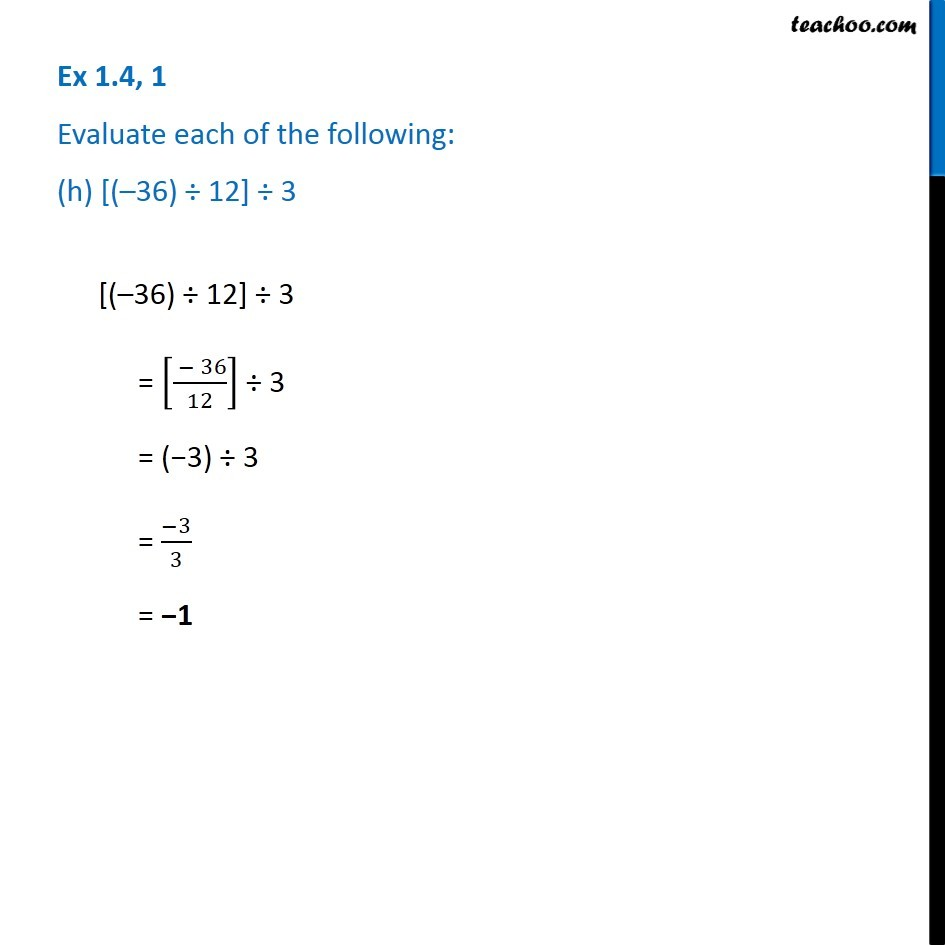1. Chapter 1 Class 7 Integers
2. Serial order wise
3. Ex 1.4

Transcript

Ex 1.4, 1 Evaluate each of the following: (h) [( 36) 12] 3 [( 36) 12] 3 = [( 36)/12] 3 = ( 3) 3 = ( 3)/3 = 1

Ex 1.4

Chapter 1 Class 7 Integers
Serial order wise